## Time and Work: Formulas, Tricks, Examples and Online Test

Time and Work is one of the important topics of competitive exams. Questions are often being asked in this topic in the above competitive exams. We have already learned this topic in our lower classes. Even though we have already learned this topic in our lower classes, we need to learn some more tricks which are being used to solve the problems on the above topic. The only thing we have to do is, we have to apply the appropriate tricks and solve the problems in a limited time. This limited time would be one minute or less in most of this type of exams.

In most of the problems on time and work, either of the following basic parameters are to be calculated:

### Quicker Method to solve the Questions

If a person can do a piece of work in ‘m’ days, he can do (1/m) part of the work in 1 day.

Example 1: Ram will do a piece of work in 15 days, what part of work will he do in two days?

Solution: Here, Man × Days = Man days

1 × 15 = 15, as given work will take 15 days

Therefore in one day,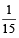th of the work will be done

and in 2 days,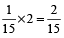of the work will be done.

If the number of persons engaged to do a piece of work be increased (or decreased) in a certain ratio the time required to do the same work will be decreased (or increased) in the same ratio.

If A is twice as good a workman as B, then A will take half the time taken by B to do a certain piece of work.

Example 2: Ram is twice as good as Shyam in work; Shyam will do a piece of work in 30 days, in how many days Ram will do the work?

Solution: Since Ram is twice as good, he will do the work in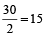days

#### Time and work are always in direct proportion.

If A and B can do a piece of work in X and Y days respectively while working alone, they will together take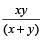days to complete it.

Proof: A’s 1 day of work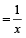B’s 1 day of work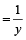So (A + B) ’s total 1 day work =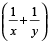of the total work.

Let total work be W.

Now,of W can be finished in 1 day

W (total work) can be finished in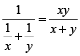days.

Example 3: Shyam will do a piece of work in 30 days; Ram can do same work in 15 days, in how many days can both do the work?

Solution: As per the formula, required days

=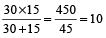days

If A , B , C can do a piece of work in X , Y , Z days respectively while working alone , they will together take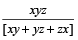days to finish it

Example 4: Shyam will do a piece of work in 30 days; Ram can do same work in 15 days, Bhuvan can do the same work in 10 days, in how many days can all three do the work?

Solution: As per the formula, required days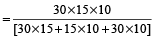=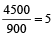days

If A can finish work in X days and B in Y days and A, B and C together in S day then :

C can finish work alone in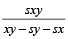B + C can finish in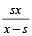and

A + C can finish in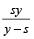Example 5: A and B together can do a piece of work in 6 days and A alone can do it in 9 days. In how many days can B alone do it?

Solution: (A + B) ’s 1 day’s work

=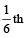part of the whole work.

A’s 1 day’s work

=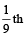part of the whole work.

∴ B’s 1 day’s work

=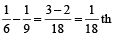part of the whole work.

∴ B alone can do the work in 18 days.

By Direct Formula:

B alone can do the whole work in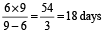Example 6: A and B can do alone a job in 6 days and 12 days. They began the work together but 3 days before the completion of job, A leaves off. In how many days will the work be completed?

1. 6 days
2. 4 days
3. 5 days
4. 7 days

Solution: (1): Let work will be completed in x days. Then,

work done by A in (x – 3) days + work done by B in x days = 1

i.e.,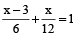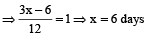By Direct Formula: Required time

=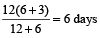Example 7: A is half good a workman as B and together they finish a job in 14 days. In how many days working alone will B finish the job.

1. 20 days
2. 21 days
3. 22 days
4. None of these

Solution: (2): Let B can do the work in x days

and A can do the work in 2x days

Then,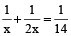(given)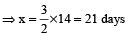By Direct Formula:

Time taken by B =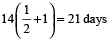Example 8: 10 men can finish a piece of work in 10 days, where as it takes 12 women to finish it in 10 days. If 15 men and 6 women undertake to complete the work, how many days they will take to complete it?

1. 7 days
2. 5 days
3. 4 days
4. 6 days

Solution: (2) It is clear that 10 men = 12 women or 5 men = 6 women

⇒ 15 men + 6 women = (18 + 6) i.e. 24 women

Now 12 women can complete the work in 10 days

∴ 24 women will do it in 5 days.

By Direct Formula:

Required time =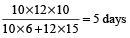If ‘M₁’ persons can do ‘W₁’ works in ‘D₁’ days and ‘M₂’ persons can do ‘W₂’ works in ‘D₂’ days then

M₁ D₁ W₂ = M₂ D₂ W₁

If T₁ and T₂ are the working hours for the two groups then

M₁ D₁ W₂ T₁ = M₂ D₂ W₁ T₂

Similarly, M₁ D₁ W₂ T₁ E₁ = M₂ D₂ W₁ T₂ E₂,

Where E₁ and E₂ are the efficiencies of the two groups.

If the number of men to do a job is changed in the ratio a : b, then the time required to do the work will be in the ratio b : a, assuming the amount of work done by each of them in the given time is the same, or they are identical.

A is K times as good a worker as B and takes X days less than B to finish the work. Then the amount of time required by A and B working together is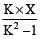days.

If A is n times as efficient as B, i.e. A has n times as much capacity to do work as B, A will take 1/n of the time taken by B to do the same amount of work.

Example 9: 5 men prepare 10 toys in 6 days working 6 hrs a day. Then in how many days can 12 men prepare 16 toys working 8 hrs a day?

Solution: This example has an extra variable ‘time’ (hrs a day) , so the ‘basic-formula’ can’t work in this case. An extended formula is being given :

M₁ D₁ T₁ W₂ = M₂ D₂ T₂ W₁

Here, 5 × 6 × 6 × 16 = 12 × D₂ × 8 × 10

∴ D₂ =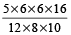= 3 days.